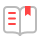<p style="margin: 0px; padding: 0px; line-height: 30px; text-indent: 28px; font-family: " pingfang="" sc",="" "hiragino="" sans="" gb",="" stheiti,="" "microsoft="" yahei",="" jhenghei",="" "source="" han="" "noto="" cjk="" cn",="" tc",="" "wenquanyi="" micro="" hei",="" simsun,="" sans-serif;="" color:="" rgb(64,="" 64,="" 64);"="">